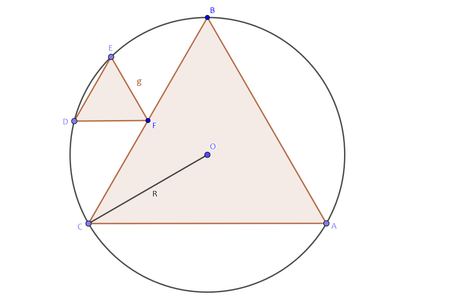# Practice on Toph

Participate in exhilarating programming contests, solve unique algorithm and data structure challenges and be a part of an awesome community.

# Sakuru Sankakkei

By mohibur · Limits 1s, 512 MB

Shawon and Tarek are very good friends. One day Shawon gives his friend Tarek a geometry problem. Tareq is very good at geometry and he solved the problem easily.
Shawon will be very happy if you solve this problem too. Can you solve this problem?You are given a circle with radius R. Inside the circle there is an equilateral triangle ABC. There is another equilateral triangle DEF where F is the midpoint of side BC. You have to find out the AREA of the triangle DEF. For better understanding, see the picture above.

Constraints:

1 ≤ T ≤ $10^{3}$

1 ≤ R ≤ $10^{5}$

## Input

Input starts with an integer T denoting the number of test cases. Each case contains one integer R, radius of the circle.

## Output

For each case of input, print the case number and then print the area of the triangle DEF. Print the answer with exactly 2 digits after the decimal point.

## Sample

InputOutput
2
10
12

12.40
17.86


### Statistics

75% Solution Ratio

steinumEarliest, 6M ago

RubiathFastest, 0.0s

steinumLightest, 131 kB

steinumShortest, 320B

### Submit